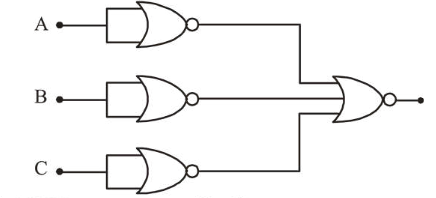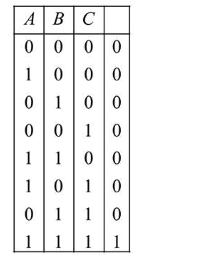# Identify the operation performed by the circuit given below:

Question:

Identify the operation performed by the circuit given below:1. NAND

2. OR

3. AND

4. NOT

Correct Option: , 3

Solution:

(3) When two inputs of NAND gate is shorted, it behaves like a NOT gate so boolen equation will be

$y=\overline{\bar{A}+\bar{B}+\bar{C}}$

$y=A \cdot B \cdot C$Thus, whole arrangement behaves like a AND gate.